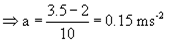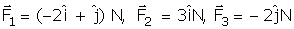NEET  >  Test: Newton’s Second Law of Motion

# Test: Newton’s Second Law of Motion

Test Description

## 10 Questions MCQ Test Physics Class 11 | Test: Newton’s Second Law of Motion

Test: Newton’s Second Law of Motion for NEET 2022 is part of Physics Class 11 preparation. The Test: Newton’s Second Law of Motion questions and answers have been prepared according to the NEET exam syllabus.The Test: Newton’s Second Law of Motion MCQs are made for NEET 2022 Exam. Find important definitions, questions, notes, meanings, examples, exercises, MCQs and online tests for Test: Newton’s Second Law of Motion below.
Solutions of Test: Newton’s Second Law of Motion questions in English are available as part of our Physics Class 11 for NEET & Test: Newton’s Second Law of Motion solutions in Hindi for Physics Class 11 course. Download more important topics, notes, lectures and mock test series for NEET Exam by signing up for free. Attempt Test: Newton’s Second Law of Motion | 10 questions in 10 minutes | Mock test for NEET preparation | Free important questions MCQ to study Physics Class 11 for NEET Exam | Download free PDF with solutions
 1 Crore+ students have signed up on EduRev. Have you?
Test: Newton’s Second Law of Motion - Question 1

### A constant force acting on a body of mass 3 kg changes its speed from 2 m/s to 3.5 m/s in 10 second. If the direction of motion of the body remains unchanged, what is the magnitude and direction of the force?

Detailed Solution for Test: Newton’s Second Law of Motion - Question 1

Given : Mass, m = 3 kg;

Initial Velocity, u = 2m/s;

Final velocity, v = 3.5 m/s

Time taken, t = 10 seconds

v = u+at

3.5 = 2 +a x 10F = ma = 3 x 0.15 = 0.45 N

Since the applied force increase the speed of the body, it acts along the direction of motion.

Test: Newton’s Second Law of Motion - Question 2

### The product of mass and velocity is known as

Detailed Solution for Test: Newton’s Second Law of Motion - Question 2

The product of velocity and mass is known as momentum which is a vector quantity.

Test: Newton’s Second Law of Motion - Question 3

### In cgs system the gravitational unit of force is gram weight (g wt).Then 1g wt (or g f) =_______ dyne.

Detailed Solution for Test: Newton’s Second Law of Motion - Question 3

For SI to CGS conversion,

1 Kg = 103 g

1 m = 102 cm

=> In CGS System,

1 N (Kg.m/s2) = 105 dynes (g.cm/s2)

=> 1 g.wt = 9.8 m/s2 x 102 cm / 1 s2 x 10-3 g x 10-2 cm / 1 s x 105 dynes

= 980 dynes (g.cm/s2)

Test: Newton’s Second Law of Motion - Question 4

The dimensional formula of Plancks’s constant and angular momentum are

Detailed Solution for Test: Newton’s Second Law of Motion - Question 4

Planck's Constant (h)  = 6.626176 x 10-34  m2 kg/s
So, Unit of planck constant=  m2 kg/s

Dimensions =M L2 T −1   ________ (1)

Angular momentum l = mvr

Where, m-mass

v-velocity

Dimensions of angular momentum  =  M L T −1 L   = M  L2  T −1  _______________ (2)
From (1) and (2).

Planck's constant and angular momentum have the same dimensions.

Test: Newton’s Second Law of Motion - Question 5

Which of the following quantity has the unit of newton-second?

Detailed Solution for Test: Newton’s Second Law of Motion - Question 5

Net external force F = dp/dt, so using dimensional analysis, unit of momentum should be the same as unit of force times unit of time which is Newton second.

Test: Newton’s Second Law of Motion - Question 6

If newton is the unit of force in MKS system then, in CGS system 1N= ______ dyne

Detailed Solution for Test: Newton’s Second Law of Motion - Question 6

1N = kg m /sec
1 dyne = gram cm/sec
Thus 1N in CGS system = 1000 gram 100 cm/sec
That is 1N = 100000 dyne

Test: Newton’s Second Law of Motion - Question 7

Three forcesact on an object of mass m = 2 kg. The acceleration of the object in m/s2 is:

Detailed Solution for Test: Newton’s Second Law of Motion - Question 7

Force vector follows the principle of superposition which says all the force vectors can be vectorially added if applied on one point to get the net force vector. Hence we get
F = F1 + F2 + F3
= (-2 + 3) i + (1 - 2) j
F = i - j = ma
Thus we get a = (i - j) /m
= (i - j) / 2

Test: Newton’s Second Law of Motion - Question 8

Newton’s second law of motion relates

Detailed Solution for Test: Newton’s Second Law of Motion - Question 8

Newton's 2nd Law of motion states that rate of change of momentum is directly proportional to external force applied. Hence, it relates rate of change of momentum to external force applied.

Test: Newton’s Second Law of Motion - Question 9

A cyclist of mass 30 kg exerts a force of 250 N to move his cycle. The acceleration is 4 ms−2. The force of friction between the road and tyres will be

Detailed Solution for Test: Newton’s Second Law of Motion - Question 9

F = ma so a= 250/30

a = 8.34

now the result is 4mt/secsqure so reduction is 4.34

so friction force will b 4.34 ×30

F is 130.2 n

Test: Newton’s Second Law of Motion - Question 10

The time rate of change of momentum is called:

Detailed Solution for Test: Newton’s Second Law of Motion - Question 10

Time rate of change of angular momentum represents torque. Hence force is known as rate change of momentum. The rate of change of momentum is called force by Newton's second law of motion.

## Physics Class 11

126 videos|449 docs|210 tests
 Use Code STAYHOME200 and get INR 200 additional OFF Use Coupon Code
Information about Test: Newton’s Second Law of Motion Page
In this test you can find the Exam questions for Test: Newton’s Second Law of Motion solved & explained in the simplest way possible. Besides giving Questions and answers for Test: Newton’s Second Law of Motion, EduRev gives you an ample number of Online tests for practice

## Physics Class 11

126 videos|449 docs|210 tests

### How to Prepare for NEET

Read our guide to prepare for NEET which is created by Toppers & the best Teachers